# 978-0073382395 Chapter 20 Questions and Problems

Document Type
Homework Help
Book Title
Fundamentals of Corporate Finance Standard Edition 9th Edition
Authors
Stephen Ross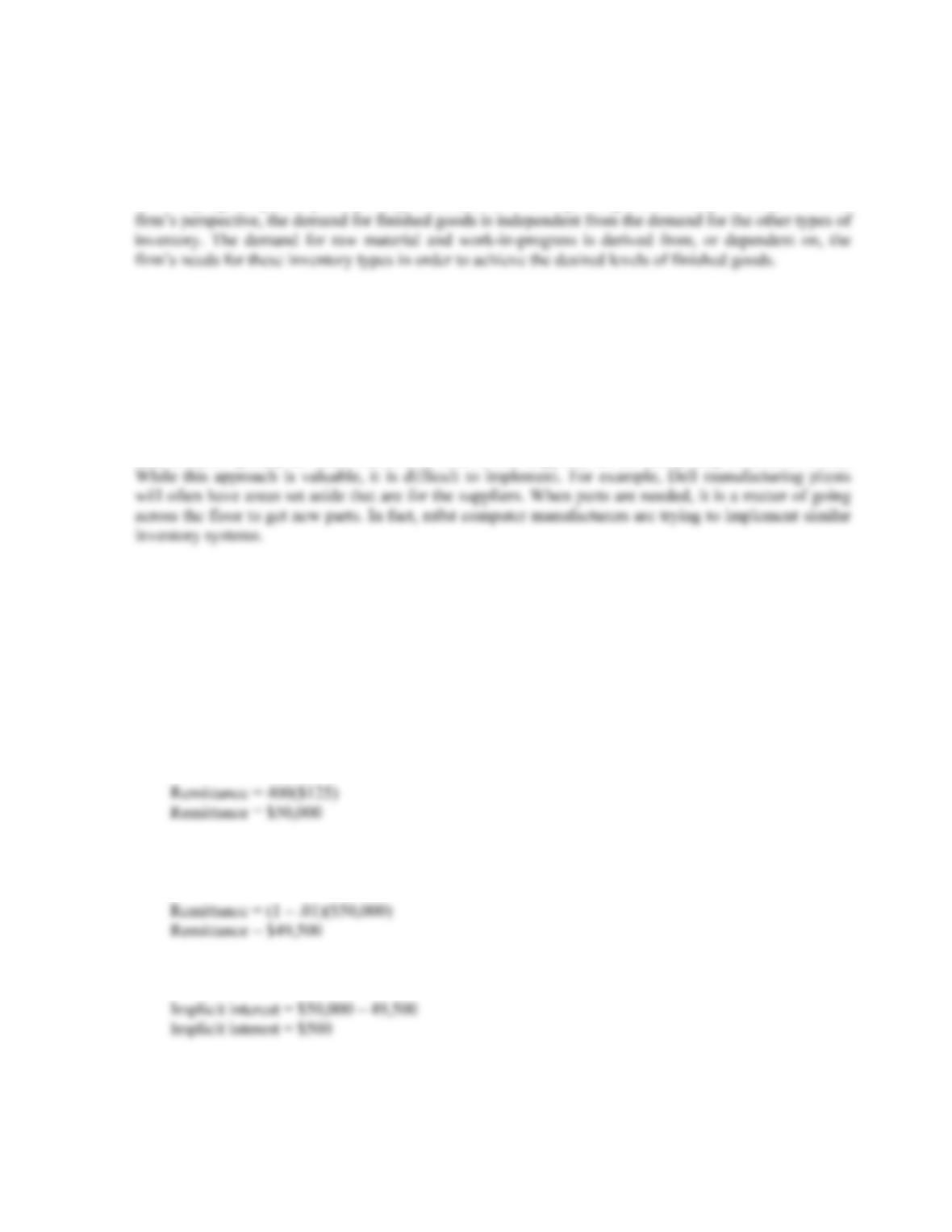CHAPTER 20 B-331
e. A: Rugs are fairly standardized and they are transportable, while carpets are custom fit and are
not particularly transportable.
7. The three main categories of inventory are: raw material (initial inputs to the firm’s production process),
work-in-progress (partially completed products), and finished goods (products ready for sale). From the
8. JIT systems reduce inventory amounts. Assuming no adverse effects on sales, inventory turnover will
increase. Since assets will decrease, total asset turnover will also increase. Recalling the DuPont
equation, an increase in total asset turnover, all else being equal, has a positive effect on ROE.
9. Carrying costs should be equal to order costs. Since the carrying costs are low relative to the order costs,
the firm should increase the inventory level.
10. Since the price of components can decline quickly, Dell does not have inventory which is purchased and
then declines quickly in value before it is sold. If this happens, the inventory may be sold at a loss.
Solutions to Questions and Problems
NOTE: All end of chapter problems were solved using a spreadsheet. Many problems require multiple steps.
Due to space and readability constraints, when these intermediate steps are included in this solutions
manual, rounding may appear to have occurred. However, the final answer for each problem is found
without rounding during any step in the problem.
Basic
1. a. There are 30 days until account is overdue. If you take the full period, you must remit:
b. There is a 1 percent discount offered, with a 10 day discount period. If you take the discount, you
will only have to remit:
c. The implicit interest is the difference between the two remittance amounts, or: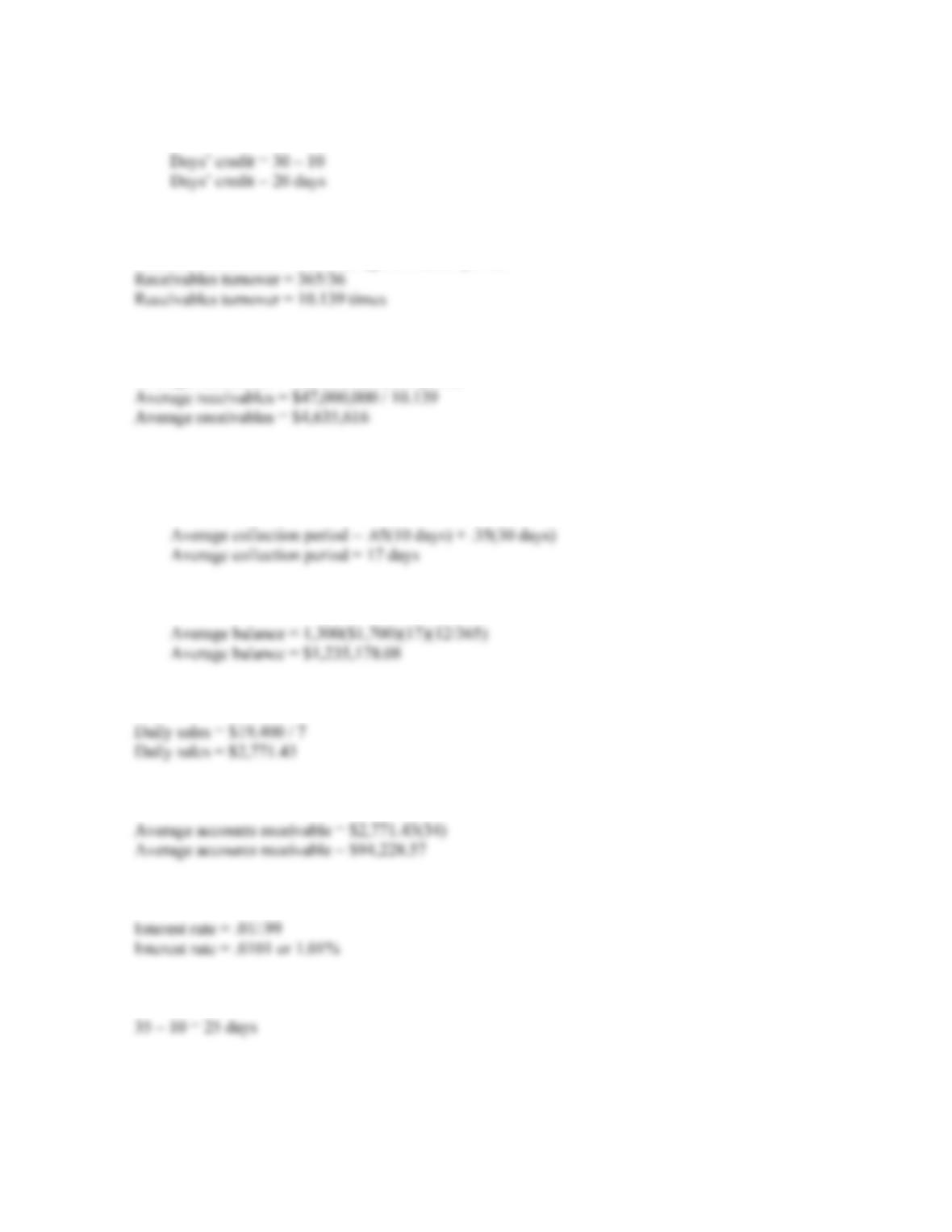B-332 SOLUTIONS
The number of days’ credit offered is:
2. The receivables turnover is:
Receivables turnover = 365/Average collection period
And the average receivables are:
Average receivables = Sales/Receivables period
3. a. The average collection period is the percentage of accounts taking the discount times the discount
period, plus the percentage of accounts not taking the discount times the days’ until full payment is
required, so:
b. And the average daily balance is:
4. The daily sales are:
Since the average collection period is 34 days, the average accounts receivable is:
5. The interest rate for the term of the discount is:
And the interest is for: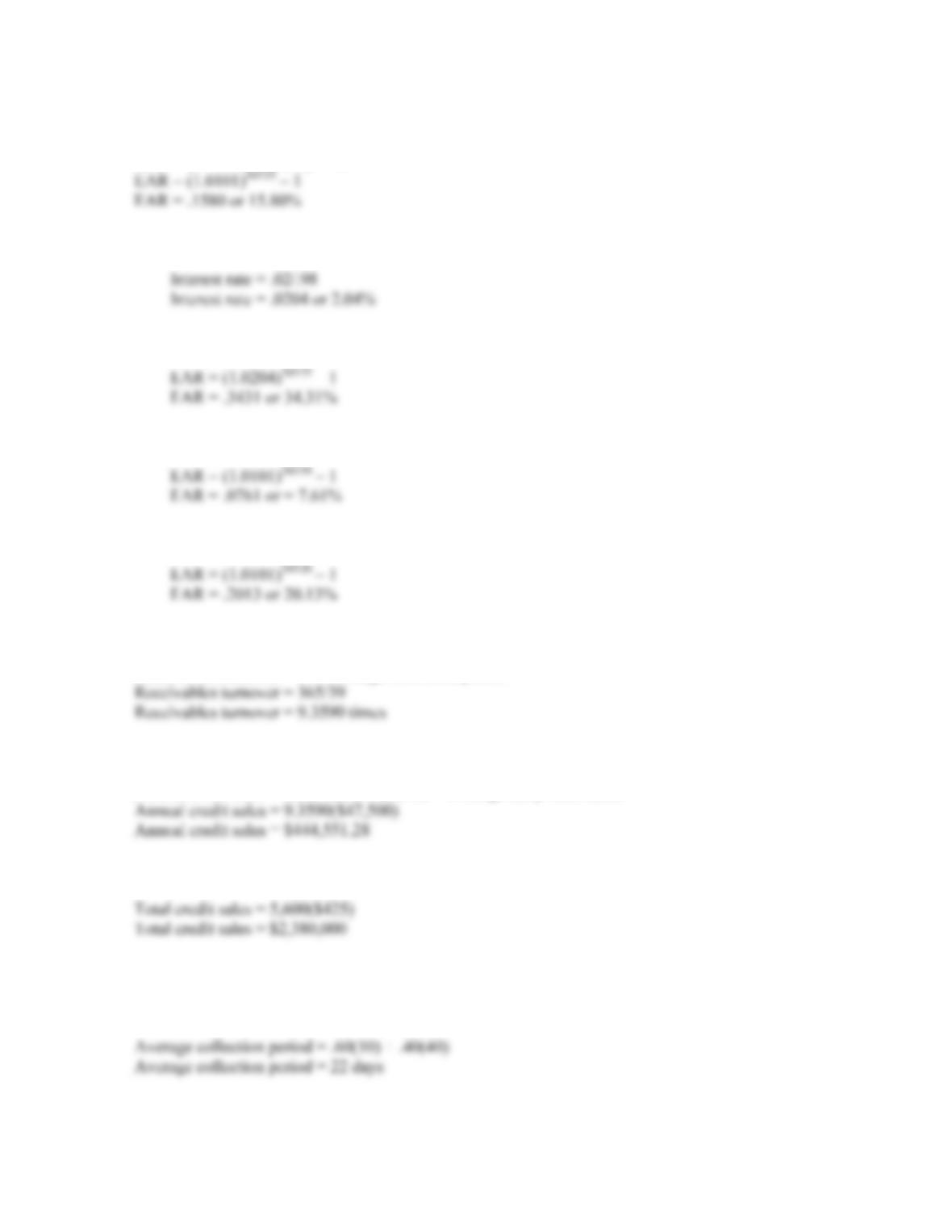CHAPTER 20 B-333
So, using the EAR equation, the effective annual interest rate is:
EAR = (1 + Periodic rate)m – 1
a. The periodic interest rate is:
And the EAR is:
b. The EAR is:
c. The EAR is:
6. The receivables turnover is:
Receivables turnover = 365/Average collection period
And the annual credit sales are:
Annual credit sales = Receivables turnover × Average daily receivables
7. The total sales of the firm are equal to the total credit sales since all sales are on credit, so:
The average collection period is the percentage of accounts taking the discount times the discount
period, plus the percentage of accounts not taking the discount times the days’ until full payment is
required, so: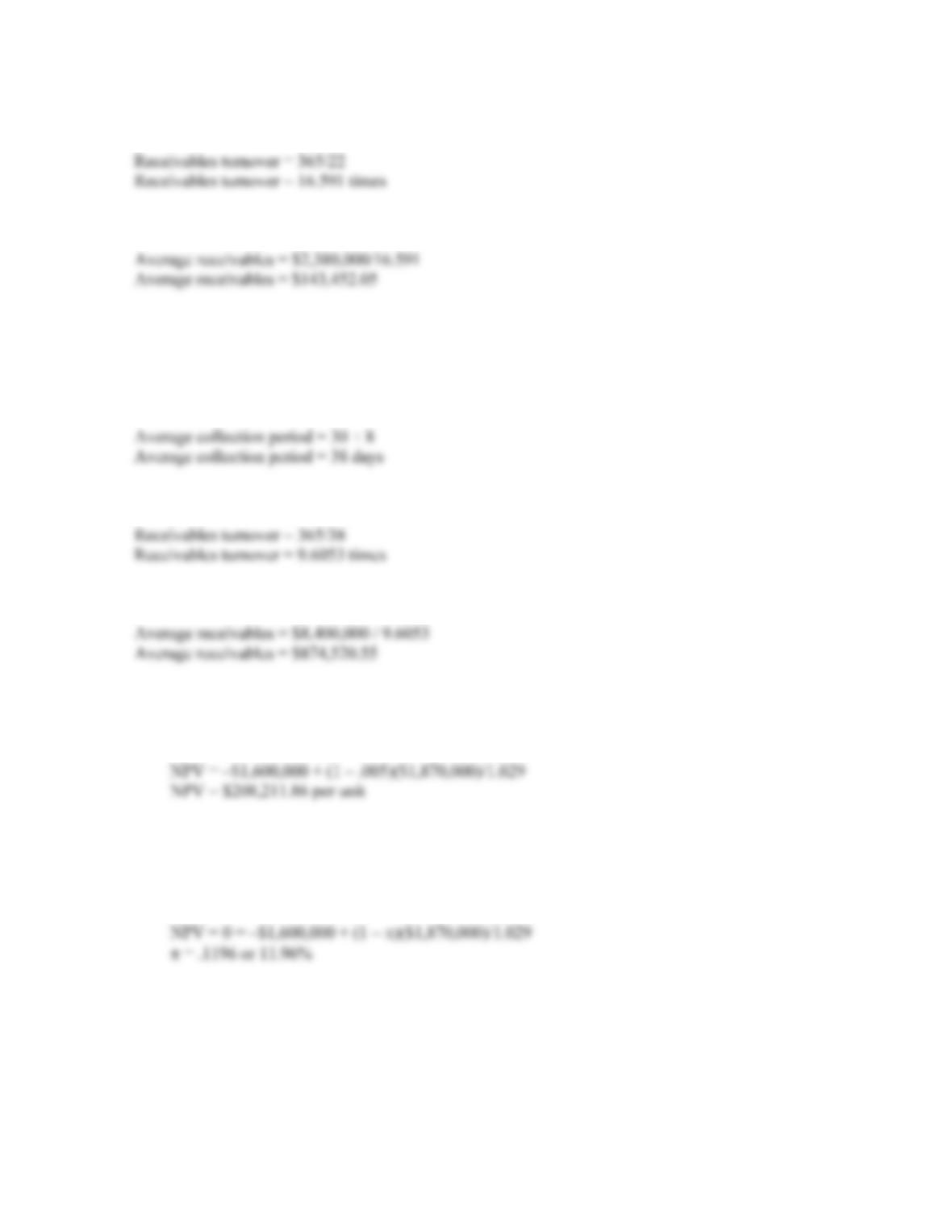B-334 SOLUTIONS
The receivables turnover is 365 divided by the average collection period, so:
And the average receivables are the credit sales divided by the receivables turnover so:
If the firm increases the cash discount, more people will pay sooner, thus lowering the average
collection period. If the ACP declines, the receivables turnover increases, which will lead to a decrease
in the average receivables.
8. The average collection period is the net credit terms plus the days overdue, so:
The receivables turnover is 365 divided by the average collection period, so:
And the average receivables are the credit sales divided by the receivables turnover so:
9. a. The cash outlay for the credit decision is the variable cost of the engine. If this is a one-time order,
the cash inflow is the present value of the sales price of the engine times one minus the default
probability. So, the NPV per unit is:
The company should fill the order.
b. To find the breakeven probability of default, , we simply use the NPV equation from part a, set it
equal to zero, and solve for . Doing so, we get:
We would not accept the order if the default probability was higher than 11.96 percent.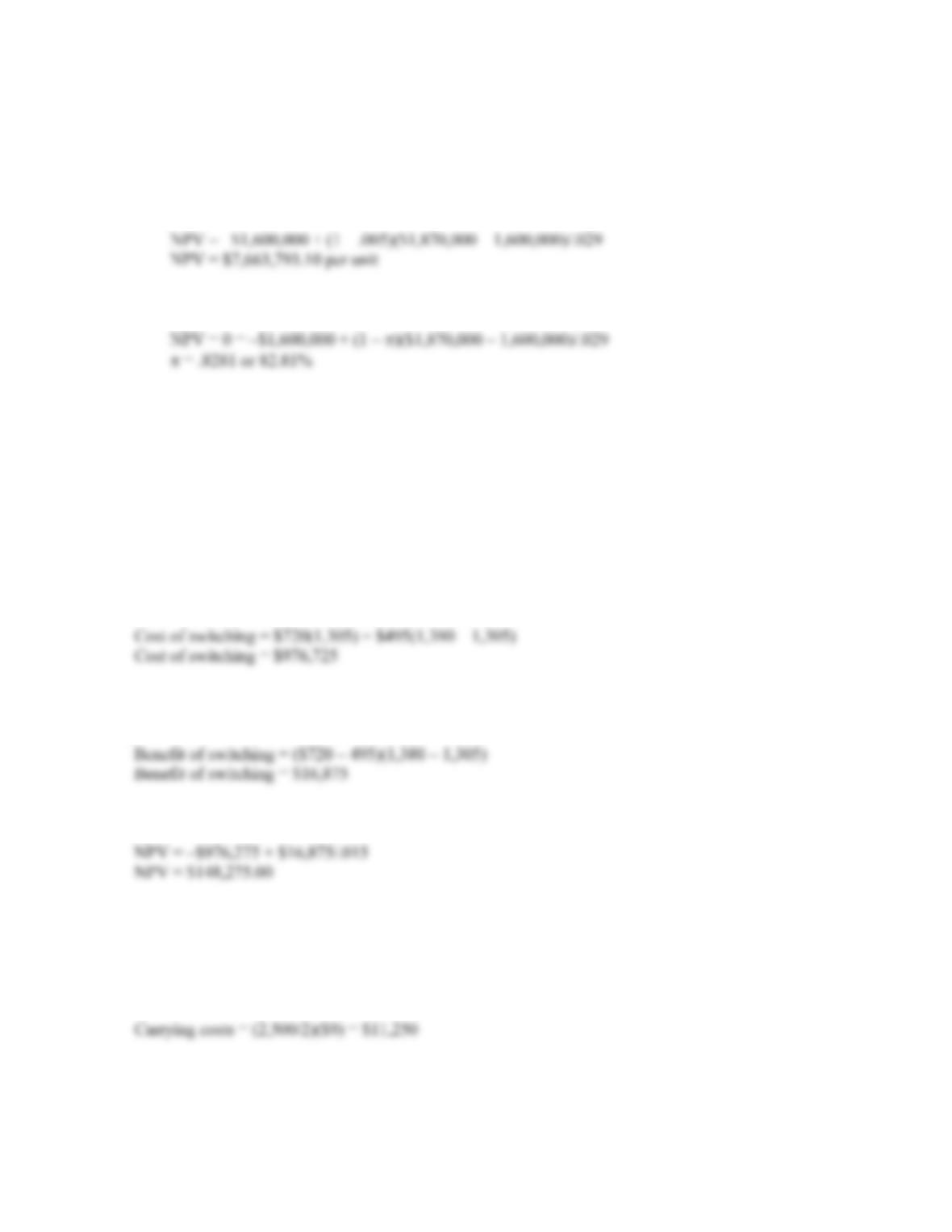CHAPTER 20 B-335
c. If the customer will become a repeat customer, the cash inflow changes. The cash inflow is now
one minus the default probability, times the sales price minus the variable cost. We need to use the
sales price minus the variable cost since we will have to build another engine for the customer in
one period. Additionally, this cash inflow is now a perpetuity, so the NPV under these assumptions
is:
The company should fill the order. The breakeven default probability under these assumptions is:
We would not accept the order if the default probability was higher than 82.81 percent. This
default probability is much higher than in part b because the customer may become a repeat
customer.
d. It is assumed that if a person has paid his or her bills in the past, they will pay their bills in the
future. This implies that if someone doesn’t default when credit is first granted, then they will be a
good customer far into the future, and the possible gains from the future business outweigh the
possible losses from granting credit the first time.
10. The cost of switching is the lost sales from the existing policy plus the incremental variable costs under
the new policy, so:
The benefit of switching is the new sales price minus the variable costs per unit, times the incremental
units sold, so:
The benefit of switching is a perpetuity, so the NPV of the decision to switch is:
The firm will have to bear the cost of sales for one month before they receive any revenue from credit
sales, which is why the initial cost is for one month. Receivables will grow over the one month credit
period and will then remain stable with payments and new sales offsetting one another.
11. The carrying costs are the average inventory times the cost of carrying an individual unit, so: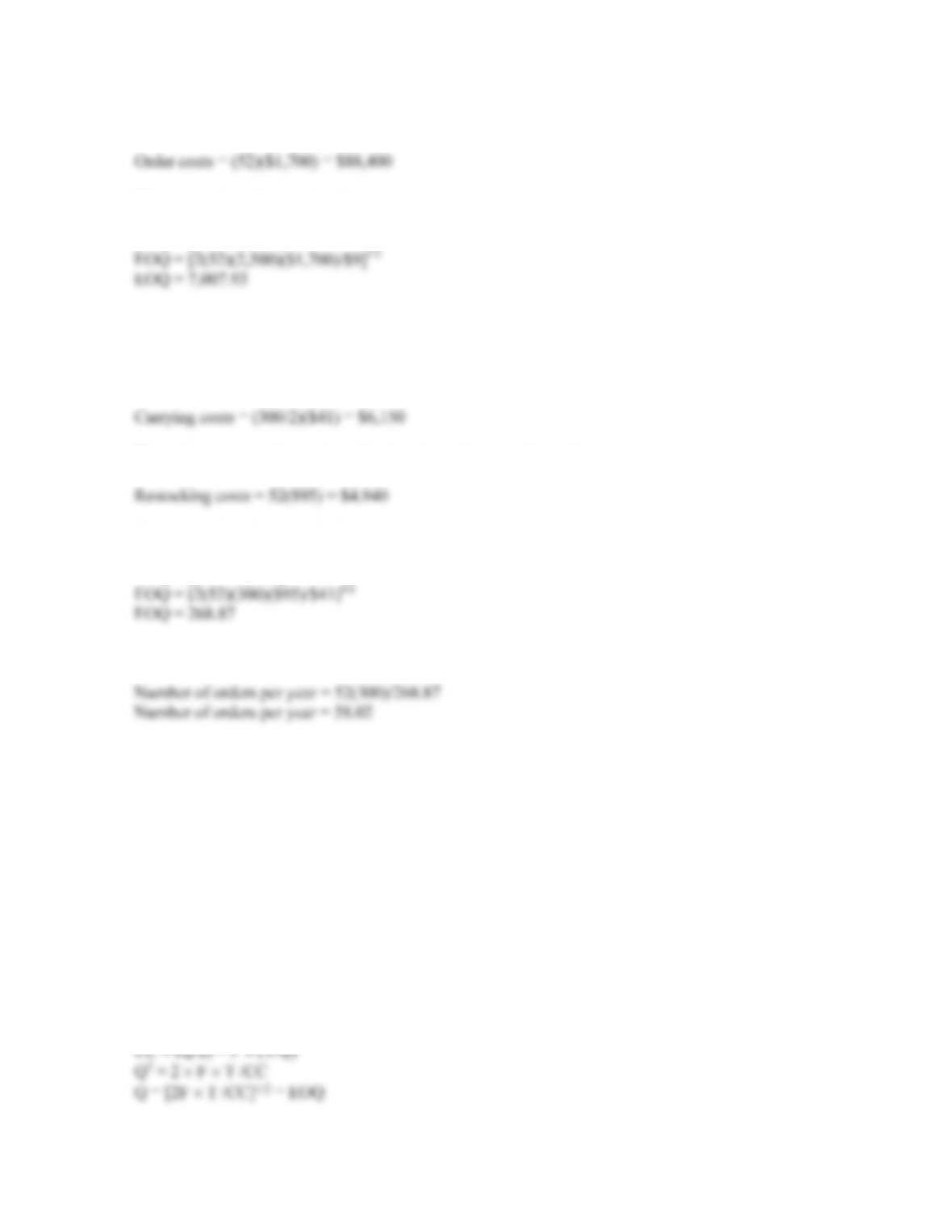B-336 SOLUTIONS
The order costs are the number of orders times the cost of an order, so:
The economic order quantity is:
EOQ = [(2T × F)/CC]1/2
The firm’s policy is not optimal, since the carrying costs and the order costs are not equal. The company
should increase the order size and decrease the number of orders.
12. The carrying costs are the average inventory times the cost of carrying an individual unit, so:
The order costs are the number of orders times the cost of an order, so:
The economic order quantity is:
EOQ = [(2T × F)/CC]1/2
The number of orders per year will be the total units sold per year divided by the EOQ, so:
The firm’s policy is not optimal, since the carrying costs and the order costs are not equal. The company
should decrease the order size and increase the number of orders.
Intermediate
13. The total carrying costs are:
Carrying costs = (Q/2) CC
where CC is the carrying cost per unit. The restocking costs are:
Restocking costs = F (T/Q)
Setting these equations equal to each other and solving for Q, we find:
CC
(Q/2) = F (T/Q)
Q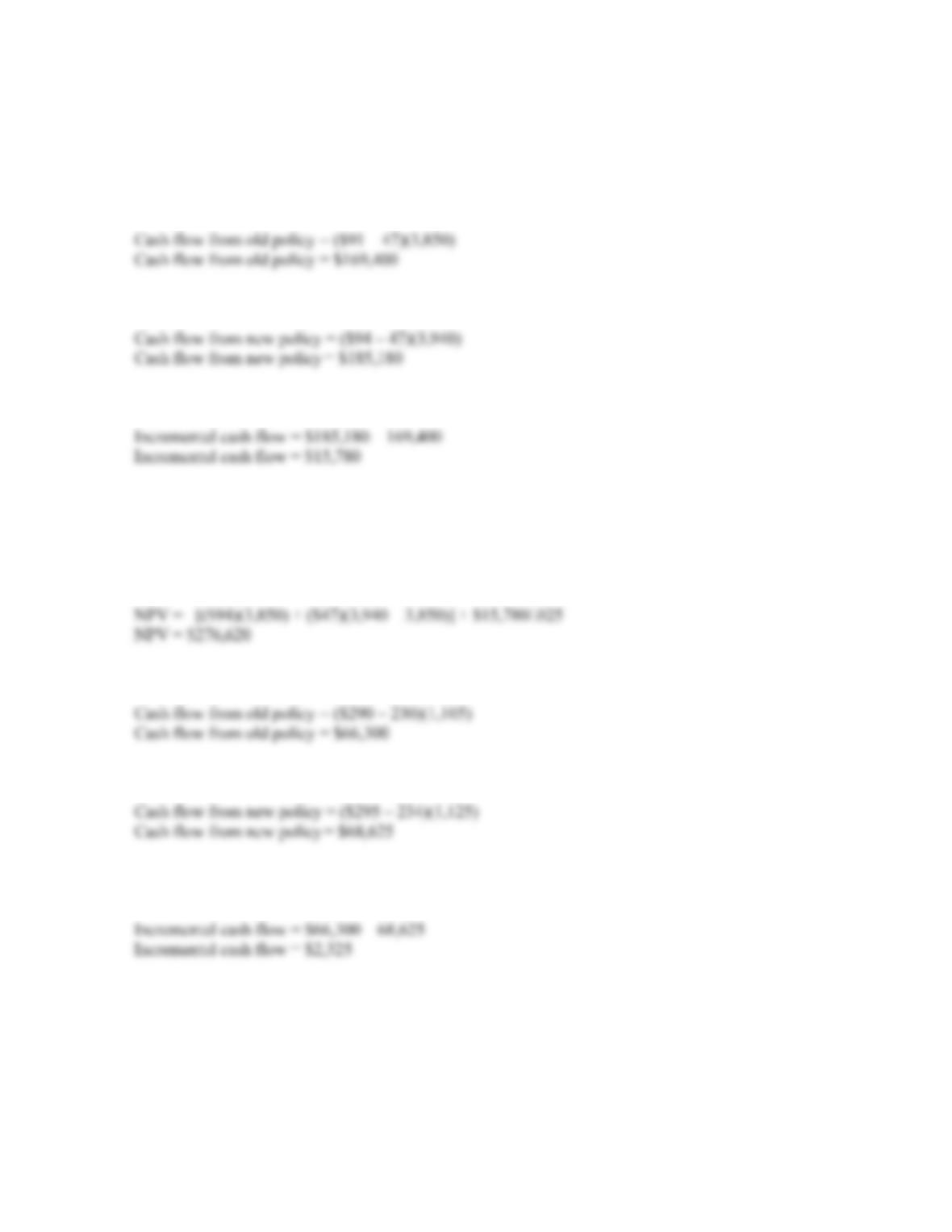CHAPTER 20 B-337
14. The cash flow from either policy is:
Cash flow = (P – v)Q
So, the cash flows from the old policy are:
And the cash flow from the new policy would be:
So, the incremental cash flow would be:
The incremental cash flow is a perpetuity. The cost of initiating the new policy is:
Cost of new policy = –[PQ + v(Q – Q)]
So, the NPV of the decision to change credit policies is:
15. The cash flow from the old policy is:
And the cash flow from the new policy will be:
The incremental cash flow, which is a perpetuity, is the difference between the old policy cash flows
and the new policy cash flows, so: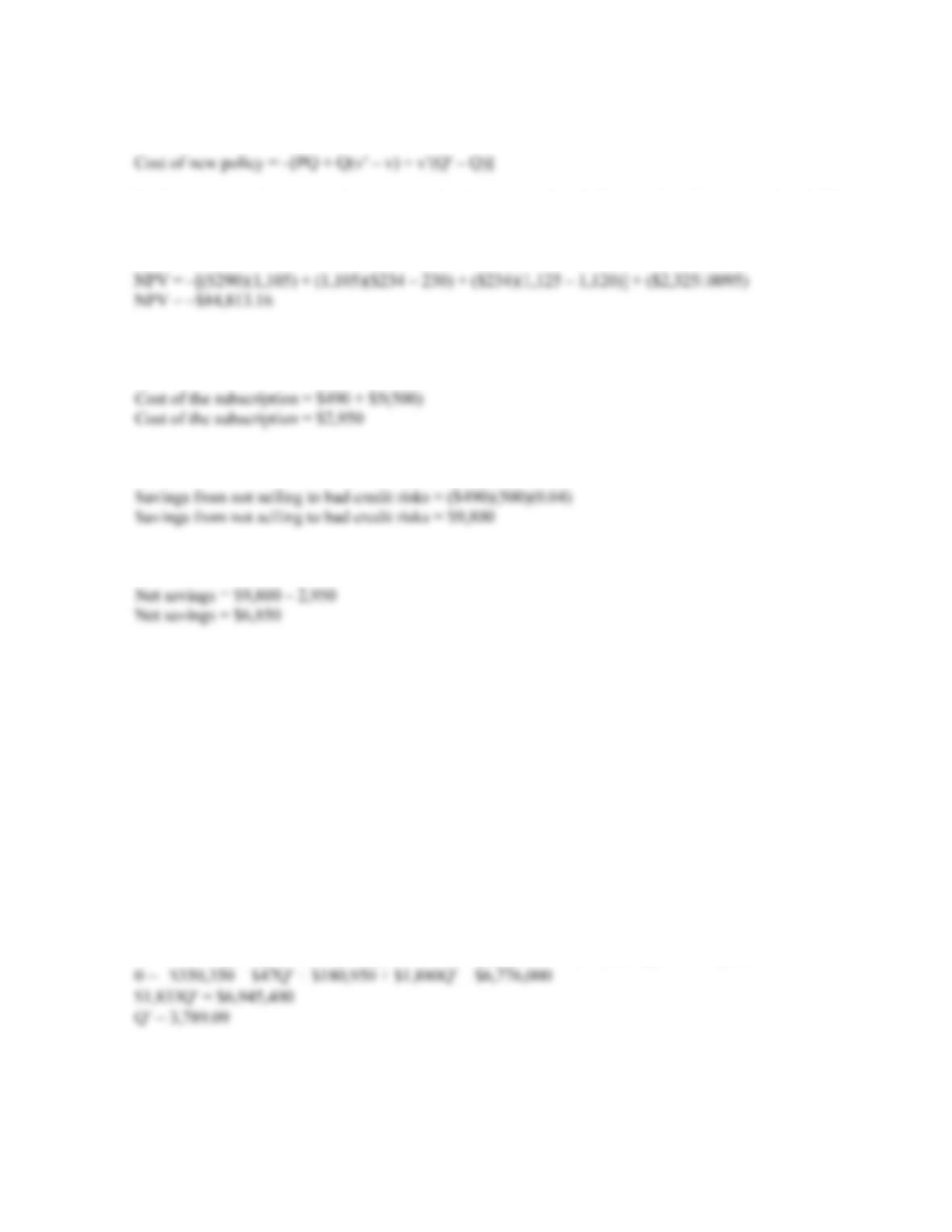B-338 SOLUTIONS
The cost of switching credit policies is:
In this cost equation, we need to account for the increased variable cost for all units produced. This
includes the units we already sell, plus the increased variable costs for the incremental units. So, the
NPV of switching credit policies is:
16. If the cost of subscribing to the credit agency is less than the savings from collection of the bad debts,
the company should subscribe. The cost of the subscription is:
And the savings from having no bad debts will be:
So, the company’s net savings will be:
The company should subscribe to the credit agency.
Challenge
17. The cost of switching credit policies is:
Cost of new policy = –[PQ + Q(v – v) + v(Q – Q)]
And the cash flow from switching, which is a perpetuity, is:
Cash flow from new policy = [Q(P v) – Q(P – v)]
To find the breakeven quantity sold for switching credit policies, we set the NPV equal to zero and
solve for Q. Doing so, we find:
NPV = 0 = –[(\$91)(3,850) + (\$47)(Q – 3,850)] + [(Q)(\$94 – 47) – (3,850)(\$91 – 47)]/.025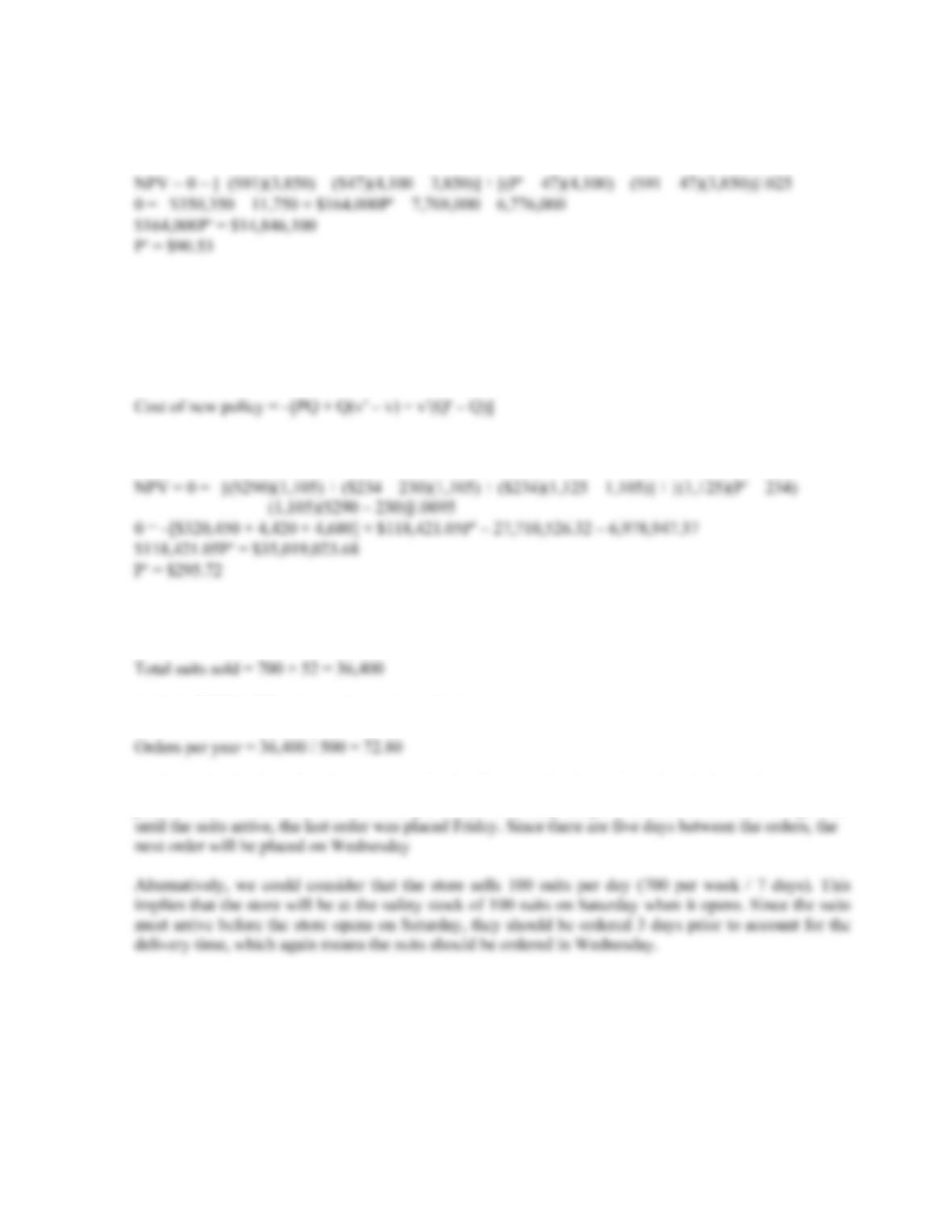CHAPTER 20 B-339
18. We can use the equation for the NPV we constructed in Problem 17. Using the sales figure of 4,100
units and solving for P, we get:
19. From Problem 15, the incremental cash flow from the new credit policy will be:
Incremental cash flow = Q(P – v) – Q(P – v)
And the cost of the new policy is:
Setting the NPV equal to zero and solving for P, we get:
20. Since the company sells 700 suits per week, and there are 52 weeks per year, the total number of suits
sold is:
And, the EOQ is 500 suits, so the number of orders per year is:
To determine the day when the next order is placed, we need to determine when the last order was
placed. Since the suits arrived on Monday and there is a 3 day delay from the time the order was placed

## Trusted by Thousands ofStudents

Here are what students say about us.